A 4x4 keypad that uses mechanical switches with individually backlit keys powered by an Arduino Pro Micro.

IntermediateFull instructions provided4 hours6,237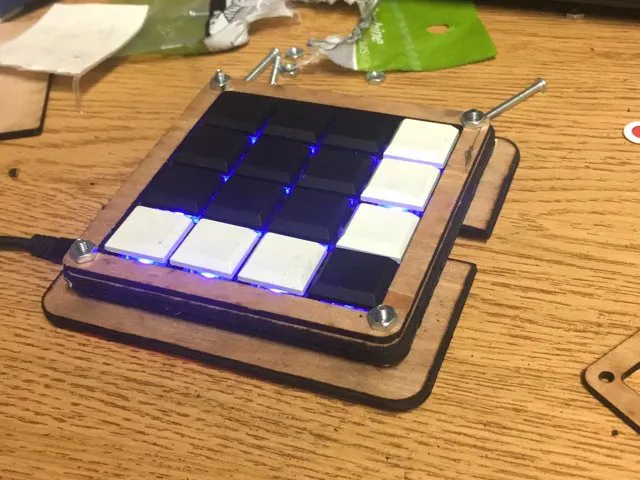## Things used in this project

### Hardware components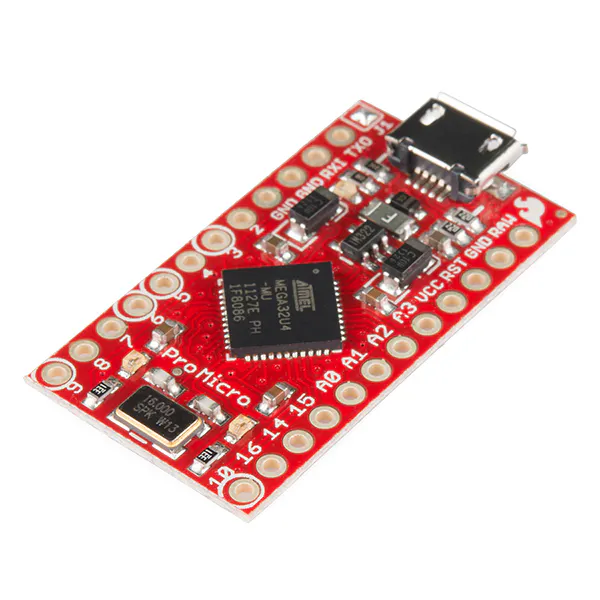SparkFun Pro Micro - 5V/16MHz
×1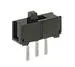Slide Switch
×3
 Texas Instruments TLC5940 TSSOP Package
×1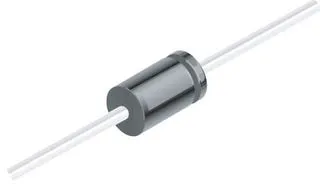1N4007 – High Voltage, High Current Rated Diode
×16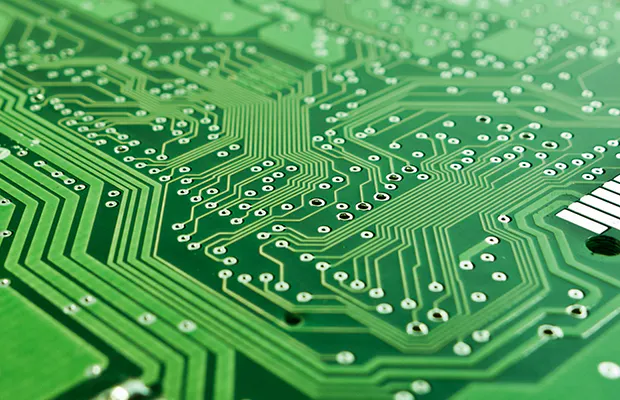Custom PCB From JLCPCB.com
×1
 Kailh Choc Low Profile Switches
×16
 Kailh Pcb Sockets
×16
 Kailh Low Profile Keycaps - Blank
×16Audio / Video Cable Assembly, Headphone The cable needs to have 4 channels male to male. Search up "TRRS cable".
×1
×1

### Software apps and online servicesArduino IDE### Hand tools and fabrication machines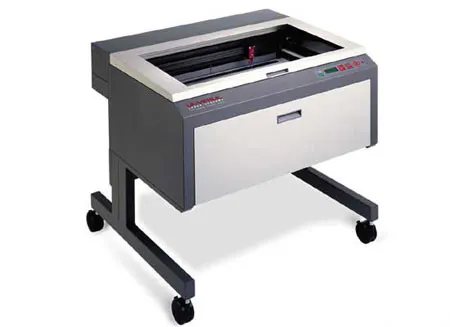Laser cutter (generic)

## Schematics

### Schematic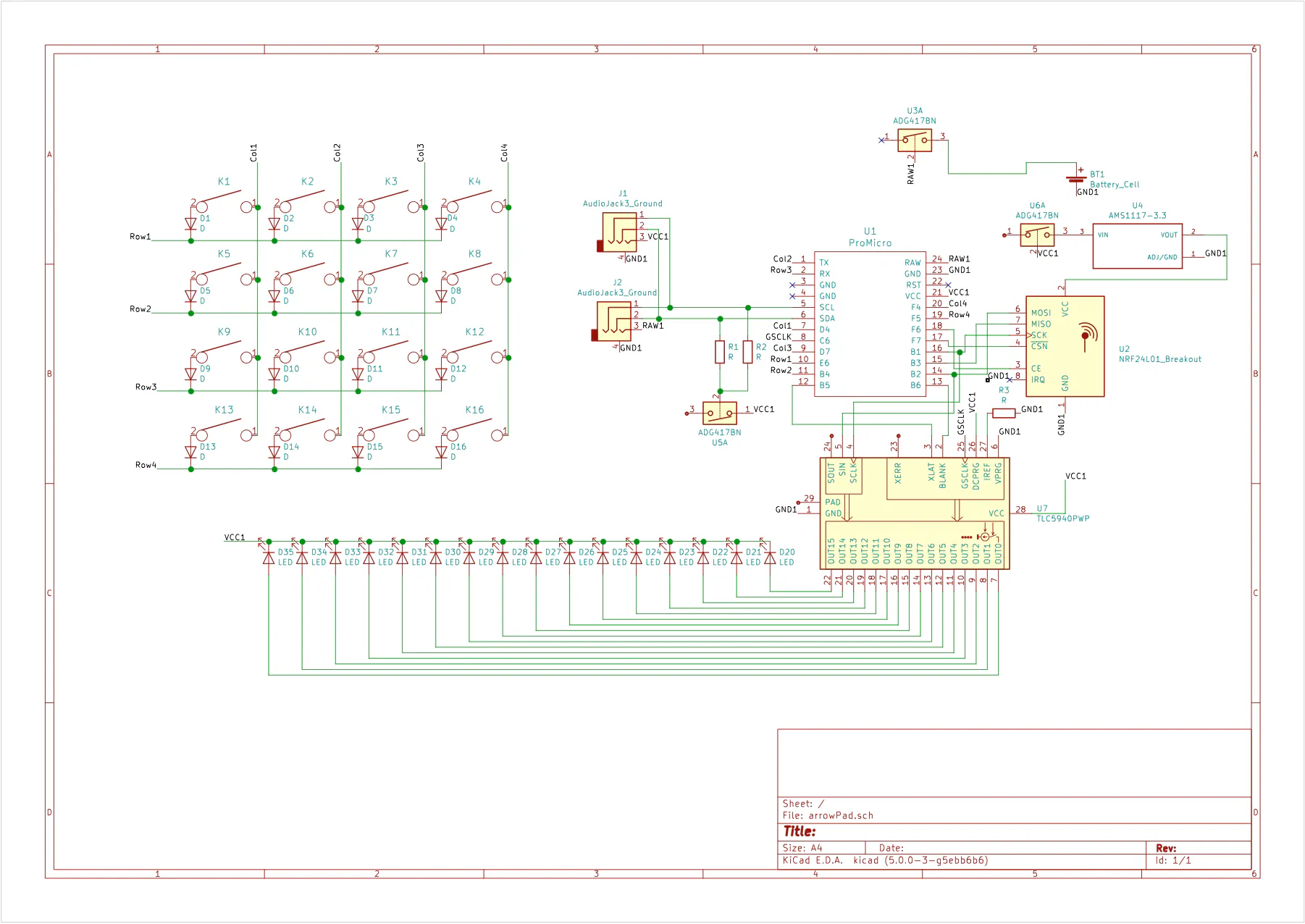## Code

### Arduino Code

Arduino
```#include <Keyboard.h>
#include "Tlc5940.h"

//byte row[] = {7, 8, 0, 20} ;//col grounds
byte row[] = {20,0,8,7};
byte col[] = {21,6,1,4};
//byte col[] = {4, 1, 6, 21} ;//input pullups
byte rowSize = sizeof(row);
byte colSize = sizeof(col);
byte keysState;

//Edit this
//change the key for each switch presse
//refer to https://www.arduino.cc/en/Reference/KeyboardPress
//modifier keys - https://www.arduino.cc/en/Reference/KeyboardModifiers
char keys =  {{'q', 'w', 'e', 'r'},
{'a','s','d','f'},
{'z', 'x', 'c', 'v'},
{NULL, NULL, NULL, ' '}};

//ledMap refers to the top left. ledMap refers to the next one
int ledMap[] = {7,6,4,5,11,10,9,8,15,14,13,12,0,1,2,3};

void setup() {
Serial.begin(9600);
Keyboard.begin();
initalize(); //sets up pins
Tlc.init(0);
}

void loop() {
//Serial.println(millis());
pressKeys(); //sends keypress to device
checkLightMode();
runLighting();
//printState(); //for debugging
delay(5); //to not over load the arduino
}

//KEYBOARD ROUTINES

for(int i = 0; i < rowSize; i++){ //iterate over each row
pinMode(row[i], OUTPUT);
digitalWrite(row[i], LOW); //allows current to flow through row
for(int j = 0; j < colSize; j++){ //interate over each switch in the row
pinMode(col[j], INPUT_PULLUP); //open the column pin up for reading
keysState[j][i] = digitalRead(col[j]); //checks the state of the switch in the row
//and stores it in keysState
pinMode(col[j], INPUT);//closes the switch for reading
} //runs until it has checked all switches in the row
//finishes checking the row
pinMode(row[i], INPUT); //stop current from flowing through the row
//move to the next row
}
}

//sends key presses
void pressKeys(){
//interate over the keyState array
for(int i = rowSize - 1; i >= 0; i--){
for(int j = colSize - 1; j >= 0; j--){
//switch at current point is pressed
if(keysState[j][i] == 0){
//Serial.print("Pressed: ");
//Serial.println(keys[i][j]);
//sends pressed value based keys array
Keyboard.press(keys[i][j]);
}

else{ //switch at current point is not pressed
//Serial.print("Released: ");
//Serial.println(keys[i][j]);
//sends release value based on keys array
Keyboard.release(keys[i][j]);
}
}
}
Serial.println();
}

void  initalize(){
//resets all pins
for(int i = 0; i < rowSize+ 1; i++){
pinMode(row[i], INPUT);
}
for(int i = 0; i < colSize; i++){
pinMode(col[i], INPUT_PULLUP);
}
}

//prints the keysState array
void printState(){
for(int i = 0; i < rowSize; i++){
Serial.print(i);
Serial.print("   ");
for(int j = 0; j < colSize; j++){
Serial.print(keysState[j][i]);
}
Serial.println();
}
}

//LIGHTING ROUTINES

int ledMode = 0;
//1 = Light pressed
//2 = Light all except pressed

//Detects key presses to change light mode
void checkLightMode(){
if(keysState == 0){
ledMode = 0;
}
else if(keysState == 0){
ledMode = 1;
}

else if(keysState == 0){
ledMode = 2;
}
}

//Chooses lighting pattern
void runLighting(){
switch(ledMode){
break;
case 1: lightReact(0);
break;
break;
}
}

//SETS Column c to specific value
void setCol(int c, int val){
for(int i = c; i <= 16; i+=4){
Tlc.set(ledMap[i], val);
}
}
//Sets all LEDS to specific value
void setAll(int x){
for (int i = 0; i < 16; i++) {
Tlc.set(i, x);
}
}

//LIGHTS: FADES COLUMNS IN WAVE PATTERN
int current1 = 500; //edit the currenti values for offsets
int current2 = 1000;
int current3 = 2000;
int current4 = 3000;
int increment5 = 100; //edit to slow or speed wave

int inc1 = 1;
int inc2 = 1;
int inc3 = 1;
int inc4 = 1;

if(current1<=0) inc1 = 1;
else if(current2<=0) inc2 = 1;
else if(current3<=0) inc3 = 1;
else if(current4<=0) inc4 = 1;
else if(current1 >=4000-increment5) inc1 = -1;
else if(current2 >=4000-increment5) inc2 = -1;
else if(current3 >=4000-increment5) inc3 = -1;
else if(current4 >=4000-increment5) inc4 = -1;
current1+= inc1*increment5;
current2+= inc2*increment5;
current3+= inc3*increment5;
current4+= inc4*increment5;
setCol(0, current1);
setCol(1, current2);
setCol(2, current3);
setCol(3, current4);
Tlc.update();
}

//LIGHTS: Fade whole board in and out

int current = 0;
int increment = 25; //edit to slow or speed the wave
int inc = increment;

current += inc;
if(current <= 0){
inc = increment;
}
else if(current >= 4000-increment){
inc = inc * -1;
}
setAll(current);
Serial.println(current);
Tlc.update();
}

//LIGHTS: REACTS TO if KEY IS PRESSED

//pres = 1 Lights all but pressed key
//pres = 0 Lights only pressed key
void lightReact(int pres){
int lightIndex = 0;
for (int i = rowSize; i < rowSize; i++){
for(int j = colSize; j < colSize; j++){
if(keysState[j][i] == pres){
Tlc.set(ledMap[lightIndex], 4000);
}
else{
Tlc.set(ledMap[lightIndex], 0);
}
lightIndex++;
}
}
Tlc.update();
}
```

## Credits

### Jonathan Low

1 project • 0 followers
I am a first-year student at UCI.